# Zeros and primes

The motivation for studying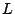-functions and their zeros is to gain information about the prime numbers. Thus, it is a fundamental problem to describe the precise connection between zeros of-functions and various properties of the prime numbers.

At present, the connection is fully understood in only a few cases, such as the equivalence between the error term in the Prime Number Theorem and the real part of the zeros of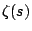. Namely,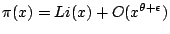is equivalent to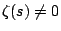for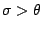.

The connection between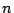-correlations of zeros of the Riemann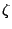-function and the distribution of the prime numbers appears to be close to being understood. See the article on the distribution of primes for a discussion.

Most of the questions about the prime numbers of a special form (twin primes, etc) haven't been shown to be related to standard conjectures about the distribution of zeros of-functions. See the articles on primes of a special form and gaps between primes for a further discussion.

The Riemann-function can be expressed as a product over the primes and also as a sum over its zeros. Thus, everything about the prime numbers can be determined from the zeros of the-function. However, not all information about the primes can be extracted from the zeros in a simple way. It is possible that some questions about the primes (eg, the variation of the distribution of primes in short intervals) are naturally related to the distribution of zeros of the Riemann-function, while other questions (twin primes?) may more naturally be related to the distribution of zeros of Dirichlet-functions. Also, some of those questions may require information beyond the GUE Hypothesis. It would be good to have some general principles describing which properties of the primes are most naturally related to which properties of particular-functions.

In this section we write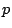for a prime and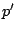for the next larger prime. We also use the standard notation for the von Mangoldt function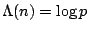if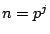and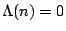otherwise, and the Chebyshev function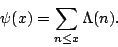Note that the prime number theorem can be written as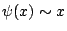.

Back to the main index for L-functions and Random Matrix Theory.